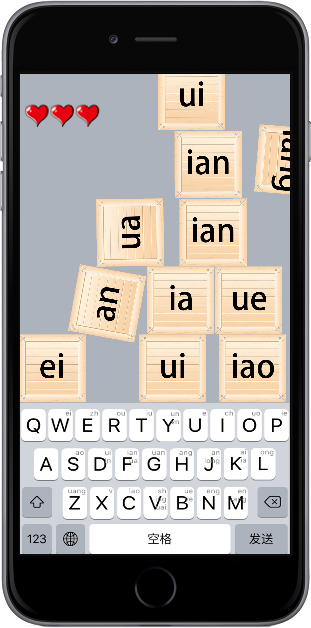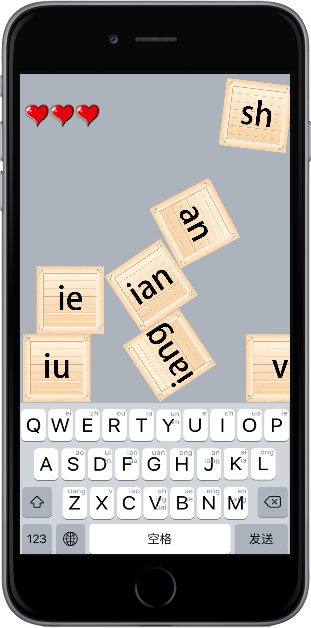# 面对coronasdk的物理引擎

local physics = require “physics” —引入物理引擎

physics.start() —启动物理引擎

physics.stop() —关闭物理引擎看到“iang”下面的部分，一个神秘的物体在箱子底下，关键是还引擎（当时我一个人晚上打代码，以为闹鬼了，吓死我了）。

main = function()

end

math.randomseed(os.time())

local seed = math.random(1,5)

choice = math.ceil(seed)

if choice == 1 then

crate = display.newImageRect(“crate1.png”,30,30)

crate.x = 120

crate.y = 5

crate.rotation = 15

physics.addBody(crate,{density = 1,friction = 0.3,bounce = 0})

elseif choice == 2 then

crate = display.newImageRect(“crate1.png”,30,30)

crate.x = 150

crate.y = 5

crate.rotation = 15

physics.addBody(crate,{density = 1,friction = 0.3,bounce = 0})

elseif choice == 3 then

crate = display.newImageRect(“crate1.png”,30,30)

crate.x = 180

crate.y = 5

crate.rotation = 15

physics.addBody(crate,{density = 1,friction = 0.3,bounce = 0})

elseif choice == 4 then

crate = display.newImageRect(“crate1.png”,30,30)

crate.x = 210

crate.y = 5

crate.rotation = 15

physics.addBody(crate,{density = 1,friction = 0.3,bounce = 0})

elseif choice == 5 then

crate = display.newImageRect(“crate1.png”,30,30)

crate.x = 240

crate.y = 5

crate.rotation = 15

physics.addBody(crate,{density = 1,friction = 0.3,bounce = 0})

end

num = num + 1

end

timer.performWithDelay(10,removeCrate,num)

“crate” is a nil value

function scene:exitScene()

–要做什麼事寫在這邊

audio.stop()

audio.dispose(backgroundMusic)

backgroundMusic = nil

background:removeSelf()

background = nil

crate1:removeSelf()

physics.stop()

local result = timer.pause(time1)

end